To view this video please enable JavaScript, and consider upgrading to a web browser that supports HTML5 video# Calculated formula questions

## 1. Introduction

Calculated Formula questions require students to make a calculation and respond with a numeric answer. The numbers change for each student and are pulled from a range that you set. The correct answer is a specific value / range of values.

You may grant partial credit for answers falling within a range. Calculated Formula questions are marked automatically. Watch this video for more information.

## 2. Process overview

The process for adding a calculated question to a test has three steps:

a. Create the question and formula.
b. Define the variables.
c. Set the answer options.

## 3. Create the question and formula

a. While creating a test, survey, or pool, hover your mouse over Create Question and click Calculated Formula.

b. Question Text must contain at least one variable. Surround variables with square brackets (e.g. [x], [y], [j] etc.). Variables are replaced by values when shown to students.

Important: Variables can't contain the letters “e,” “i” and “pi”. You can't reuse variable names. Variables can contain more than one character, such as [ab] or [cd].

c. Answer Formula is the mathematical expression used to find the correct answer. Choose operators from the top of the Answer Formula box. In the example, the formula is 4y+3x.

Important: The formula's not visible to students, MyBeckett uses it to determine the correct answer.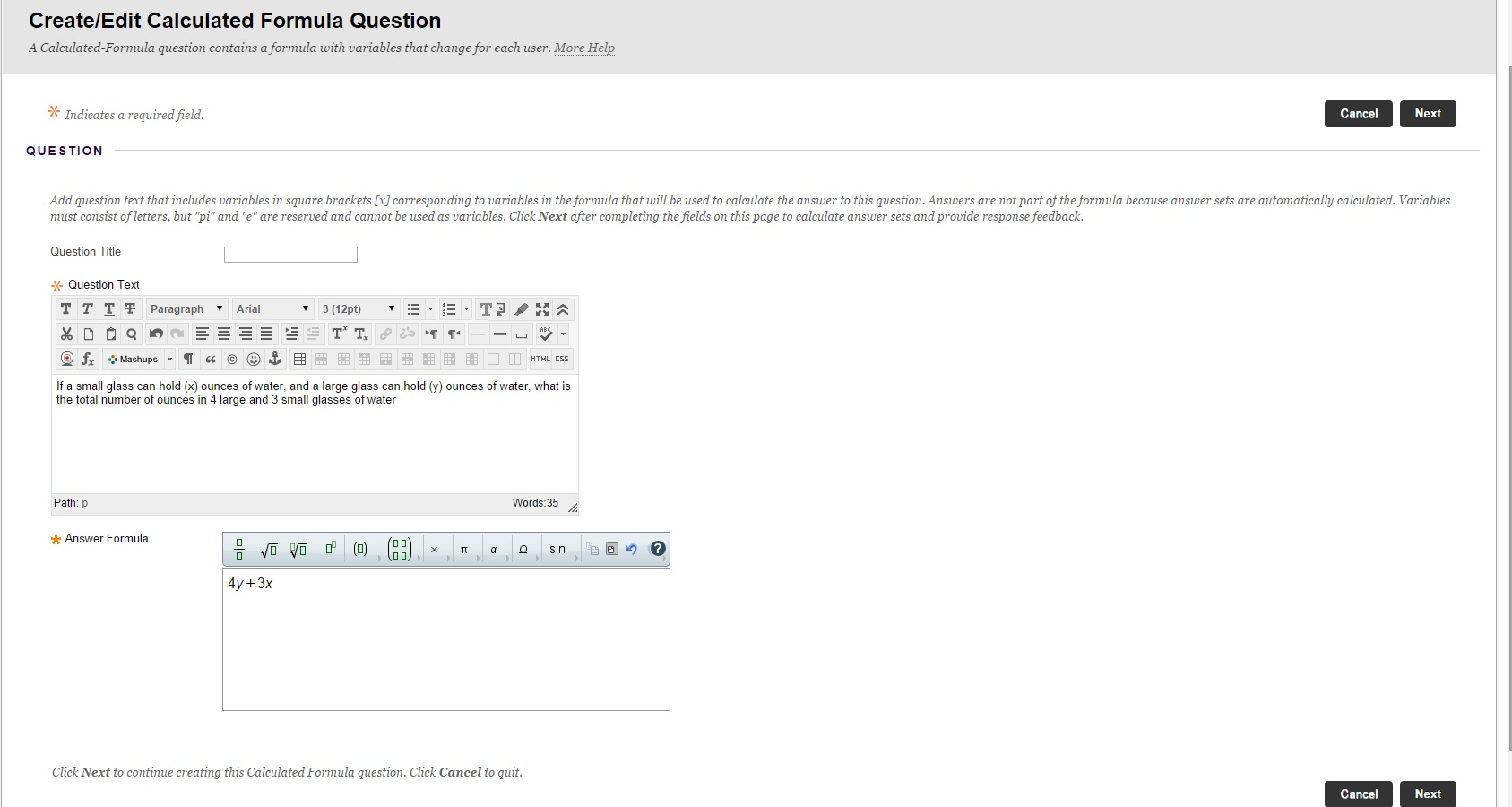d. Options, set the Answer Range to zero if the answer must be exact. Alternatively, set a range for correct answers. You can also Allow Partial Credit and select Units Required. If you do so, more options appear.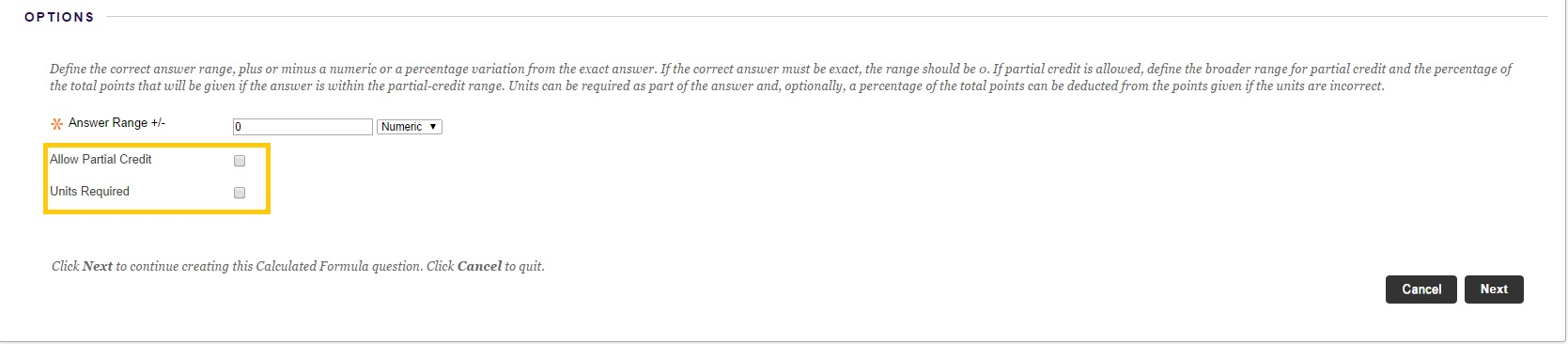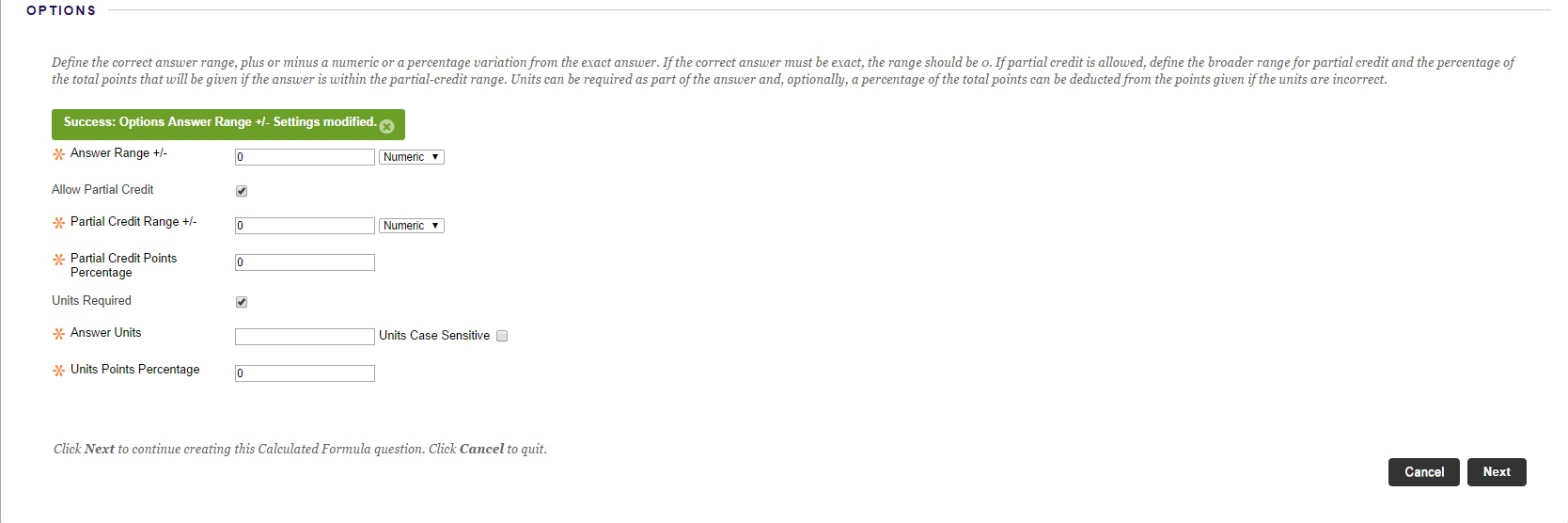In the preceding example image:

• Answers within plus or minus 4 are awarded 100% of the point total.
• Answers within partial credit range plus or minus 5 to 8 are awarded 50% of the point total.

The available options are:

• Answer Range: ;A range of answers that are awarded full credit. Choose Numeric or Percentage.
• Allow Partial Credit: Use this on a less accurate answer range. Set the Partial Credit Points Percentage to be awarded if the student’s answer is within the partial credit range.
• Units Required: The measurement unit must be provided in the student’s answer. Type the Answer Units and Units Points Percentage to be awarded if entered correctly.

e. Click Next.

## 4. Define the variables

a. Under Define Variables, set the Minimum Value and Maximum Value for each variable. When a question is presented to a student, MyBeckett replaces the variable with a value that is randomly selected from the range you defined. You can use scientific notation in the value boxes and select the number of Decimal Places for each value.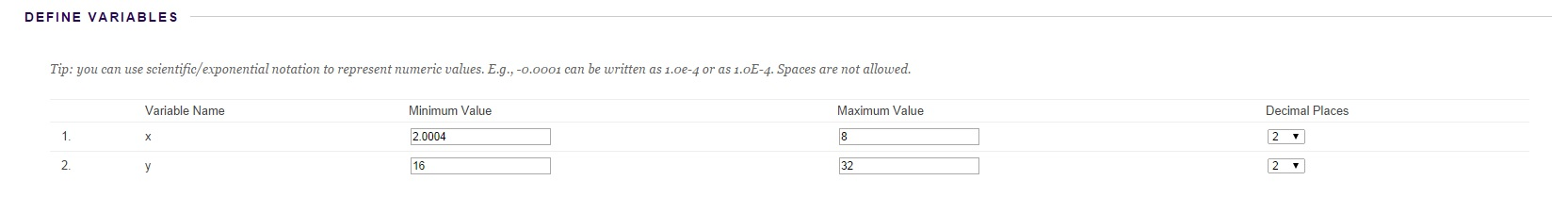Important: The number of decimal places you select can affect the minimum and maximum values of a variable. E.g. you set the minimum value as 0.0000004 and the maximum value as 1, and you choose 2 decimal places. MyBeckett rounds both numbers to 2 decimal places, therefore it generates variables with values between 0.00 and 1.00.

b. In the Answer Set Options, use the Calculate Answers to drop-down to select the number of Decimals or Significant Figures for the generated correct answers. Type the Number of Answer Sets (the number of possible variations of the question). Choose whether the Correct Answer Format is Normal or Exponential.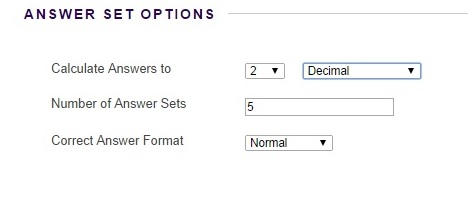c. Click. Next

## 5. Edit answer sets

You'll now see answer sets generated by the system. Each set represents one possible variation of the question that may be presented to students.

a. You can change the values of the variables in each answer set by typing in the boxes. Click Calculate to update a Calculated Answer and save changes.b. In an answer set row, click Remove to delete and automatically replace it with another set. To reduce the number of sets, click Go Back. In Answer Set Options, change the number of answer sets.

c. Optionally, type feedback for correct and incorrect answers and add metadata. You must enable feedback and metadata in Question Settings for the options to appear in questions.

d. Click Submit OR click Submit and Create Another to add the question to the test.

## Your feedback

Tell us what you think of this site

This feedback form is for web page URLs that begin with 'teachlearn' e.g. https://teachlearn.leedsbeckett.ac.uk

Page last updated: 20/02/2020